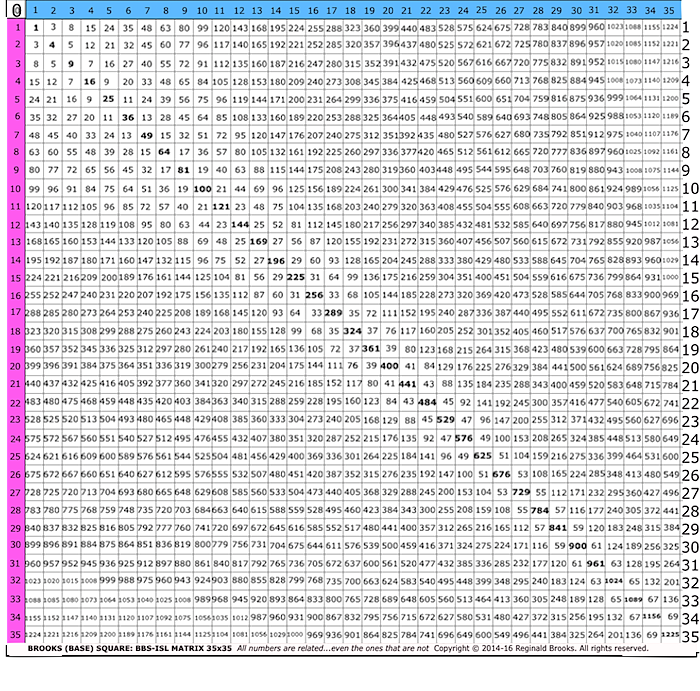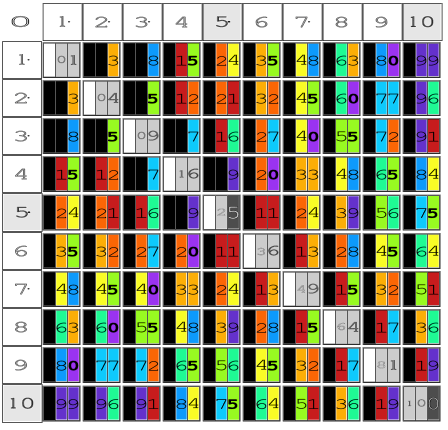# BBS-ISL Matrix

## To find the Row/Column placement of any Inner Grid ( IG) number (#):

STEPS:

1. find Factors
2. add Factors, divide by 2 = Row #
3. confirm by determining Col #
• a. divide IG # by larger Factor (or simply take the smaller Factor)
• b. subtract the resulting quotient from the Row # = Col #
• c. verify by finding the ∆ between the two PD #s

## Ex: 33 (Two Factor Sets, example for Factor Set: 3,11 only*)

1. Factors: 3, 11—(1,33)

2. Row: 3 + 11 = 14, 14 ÷ 2 = 7 = Row 7

3. Column - confirm & verify:
• a. Divide: 33 ÷ 11 = 3
• b. Subtract: 7 - 3 = 4 = Col 4
• c. verify: 7² - 4² = 49 - 16 = 33

### Therefore: IG# 33 appears 2 times on the IG at:

• Row 7, Col 4
• Row 17, Col 16 (* see note @ bottom)

## Ex: 96 (Five Factor Sets, example for four Factor Sets only)

1. Factors: 2,48—3,32—4,24—8,12—(1,96)
2. Row:
• 2 + 48 = 50, 50 ÷ 2 = 25 = Row 25
• 3 + 32 = 35, 35 ÷ 2 = 17.5 = RowXXXX (Is NOT whole integer #)
• 4 + 24 = 28, 28 ÷ 2 = 14 = Row 14
• 8 + 12 = 20, 20 ÷ 2 = 10 = Row 10
3. Column - confirm & verify:
• a. Divide:
• 96 ÷ 48 = 2
• xxx skip because not whole integer #
• 96 ÷ 24 = 4
• 96 ÷ 12 = 8
• b. Subtract:
• 25 - 2 = 23 = Col 23
• xxx
• 14 - 4 = 10 = Col 10
• 10 - 8 = 2 = Col 2
• c. verify:
• 25² - 23² = 625 - 529 = 96
• xxx
• 14² - 10² = 196 - 100 = 96
• 10² - 2² = 100 - 4 = 96

### Therefore: IG# 96 appears 4 times on the IG. The three examples at:

• Row 25, Col 23
• Row/Col XXX (skip because not whole integer #)
• Row 14, Col 10
• Row 10, Col 2
• (*see note @ bottom re: 1,96)

## SIMPLIFICATION

SIMPLIFICATION:

1. ∑ Factors ÷ 2 = Row #
2. Row # - Factor # = Col #
3. verify PD - PD = IG #

## Ex: 96 (Factors: 1,96—2,48—3,32—4,24—8,12)

### Factors: 2,48

1. ∑ Factors ÷ 2 = Row #:
• (2 + 48) ÷ 2= Row 25
2. Row # - Factor # = Col #:
• 25 - 2 = Column 23
3. verify PD - PD = IG#:
• 25² - 23² = 625 - 529 = 96

### Therefore: IG# 96 Appears on the IG at:

• Row 25, Col 23

### Factors: 3,32

1. ∑ Factors ÷ 2 = Row #:
• xxx (no IG# with this Factor Set)

### Factors: 4,24

1. ∑ Factors ÷ 2 = Row #:
• ( 4 + 24) ÷ 2= Row 14
2. Row # - Factor # = Col #:
• 14 - 4 = Column 10
3. verify PD - PD = IG#:
• 14² - 10² = 196 - 100 = 96

### Therefore: IG# 96 appears on the IG at:

• Row 14, Col 10

### Factors: 8,12

1. ∑ Factors ÷ 2 = Row #:
• ( 8 + 12) ÷ 2= Row 10
2. Row # - Factor # = Col #:
• 10 - 8 = Column 2
3. verify PD - PD = IG#:
• 10² - 2² = 100 - 4 = 96 on the IG at:
4. Row 10, Col 2

## Ex: 1125

### Factors: (25, 45)

1. ∑ Factors ÷ 2 = Row #:
• (1 + 1125) ÷ 2 = Row 563
• ( 3 + 375) ÷ 2 = Row 189
• ( 5 + 225) ÷ 2 = Row 115
• ( 9 + 125) ÷ 2 = Row 67
• ( 15 + 75) ÷ 2 = Row 45
• ( 25 + 45) ÷ 2 = Row 35
2. Row # - Factor # = Col #:
• Row 563 - 1 = Col 562
• Row 189 - 3= Col 186
• Row 115 - 5 = Col 110
• Row 67 - 9 = Col 58
• Row 45 - 15 = Col 30
• Row 35 - 25 = Col 10
3. verify by PD - PD = IG#:
• 563² - 562² = 316,969 - 315,844 = 1125
• 189² - 186² = 35,721 - 34,596 = 1125
• 115² - 110² = 13,225 - 12,100 = 1125
• 67² - 58² = 4,489 - 3,364 = 1125
• 45² - 30² = 2,025 - 900 = 1125
• 35² - 10² = 1,225 - 100 = 1125

### Therefore: IG# 1125 appears 6 times on the IG at:

• Row 563, Col 562
• Row 189, Col 186
• Row 115, Col 110
• Row 67, Col 58
• Row 45, Col 30
• Row 35, Col 10

*The Factor Set that includes 1,X where X = the IG#,
ALWAYS lies on the 1st Parallel Diagonal (3,5,7,..) if ODD;
and,if X=EVEN IG#, it will NOT be on the matrix grid, as 1+EVEN # = ODD #,
e.i. IG# 33, using Factor Set 1,33, resolves to Row 17 Col 16,
while IG# 8 does NOT have a Row/Col presence with Factor Set 1,8 as it does NOT resolve to a whole number.

## FURTHUR INSIGHTS

In the Dickson Method for calculating ALL Pythagorean Triples (PTs), factors, as Paired Factor Sets (s, t), come into play as:

• letting r=EVEN #, such that r2 = 2st is satisfied,
• gives a = r + s, b = r + t, and c = r + s + t
• and ALL PTs can be found.

In Exponentials, factors also come into play as Factor Sets (s,t) — useful for finding
the Axis Row and Column locations for any given exponential Xz value on the IG, where X = 1—2–3—… and z = 1—2—3—….

The SIMPLIFICATION method shown above is really:

• (s + t) / 2* = Row #
• Row # - s = Col. # = (t - s) / 2

## Ex: 96 (Factors:4,24)

• (s + t) / 2 = Row # = (4 + 24) / 2 = 14
• (t - s) / 2 = Col. # = (24 - 4) / 2 = 10

### Therefore: IG# 96 appears on the IG at:

• Row 14, Col 10

Furthermore, you can work back in REVERSE, if you know the IG exponential value,:

• divide t / s, until it reaches the s value,
• add the # of divisions + 1 (for the s value itself), and
• the ∑ will equal the exponential z-value of X, where X = s value.

This ONLY works for Exponential IG #s.

## Ex: 32 (Factors: 2, 16)

• 16 /2 — 8 /2—4 /2—2 /2—2 = 4 + 1 divisions = 5 = z
• X = s = 2
• Xz = 25 = 32

### Therefore: IG# 32 = 25#### ImagesKEYWORDS TAGS: TPISC, The Pythagorean - Inverse Square Connections, Pythagorean Triangles, Pythagorean Triples, primitive Pythagorean Triples, non-primitive Pythagorean Triples, Pythagorean Theorem, Pythagorus Theorem, The Dickson Method, BBS-ISL Matrix, Expanded Dickson Method, r-sets, s-set, t-sets, Pair-sets, geometric proofs, MathspeedST, leapfrogging LightspeedST FASTER than the speed of light, Brooks (Base) Square- Inverse Square Law (ISL), BBS-ISL Matrix grid, The Architecture Of SpaceTime (TAOST), The Conspicuous Absence Of Primes (TCAOP), A Fresh Piece Of Pi(e), AFPOP, Numbers of Inevitability, LightspeedST, Teachers, Educators and Students (TES), number theory, ubiquitous information, FASTER than the speed of light, primes, prime numbers, fractals, mathematics, Universe, cosmos, patterns in number.

Art Theory 101 / White Papers Index    |   New Media net.art index    |   home